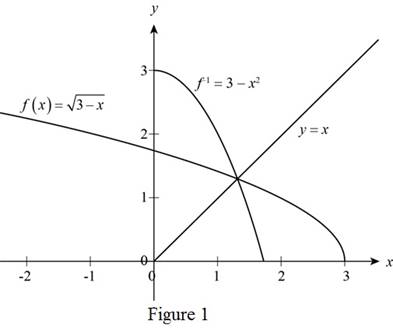# The inverse of the function f ( x ) = 3 − x### Precalculus: Mathematics for Calcu...

6th Edition
Stewart + 5 others
Publisher: Cengage Learning
ISBN: 9780840068071### Precalculus: Mathematics for Calcu...

6th Edition
Stewart + 5 others
Publisher: Cengage Learning
ISBN: 9780840068071

#### Solutions

Chapter 2, Problem 10T

(a)

To determine

## To find: The inverse of the function f(x)=3−x

Expert Solution

The inverse f1 of given function is 3x2.

### Explanation of Solution

Given:

The function is, f(x)=3x

Calculation:

Rewrite the function as y=3x (1)

The above equation is defining y as a function of x.

Square equation (1) and simplify as follows,

(y)2=(3x)2y2=3x

Isolate x on one side of the equation.

x=3y2

Interchange the variables x and y in above equation.

y=3x2

Thus, the inverse f1 of given function is 3x2.

(b)

To determine

Expert Solution

### Explanation of Solution

Use transformation and first sketch the graph of f(x)=3x

Sketch graph of x and take its reflection about y-axis and then move it right on horizontal axis by 3 units.

To sketch the graph of f1=3x2 take reflection of graph of f(x) and reflect it in the line y=x.

Sketch graph of f(x) and f1(x) as shown below.From the above Figure, it can be observed that f1(x) is a reflection f(x) in y=x.

### Have a homework question?

Subscribe to bartleby learn! Ask subject matter experts 30 homework questions each month. Plus, you’ll have access to millions of step-by-step textbook answers!